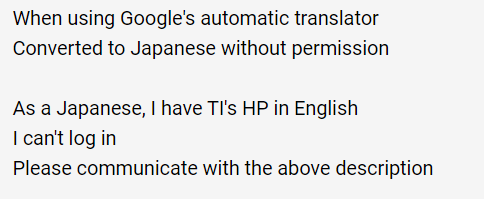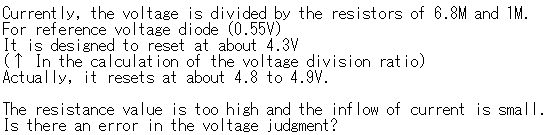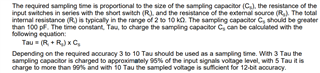If you have a related question, please click the "Ask a related question" button in the top right corner. The newly created question will be automatically linked to this question.

MSP430G2312:Voltage divider resistor (inflow current required for COMP)

Part Number: MSP430G2312

msp430g2312に関する確認

Peripheral_COMP
で私は電圧を監視したいと思います 動作は約5Vの

また、
データシートのCOMPカラム
I(DD)=45μA

• Could you use English to describe your question?

•E_COMP.txt
Confirmation regarding msp430g2312

At Peripheral_COMP
I would like to monitor the voltage
The operation is unstable with a resistance voltage divider of the mega order for a power supply of about 5V.

also,
In the data sheet COMP column
I (DD) = 45 ��A
Is it difficult unless the partial pressure is about 10K��?

Please tell me the proper digit of the voltage dividing resistor.Or the power consumption is too high?

•A1.txt
Currently, the voltage is divided by the resistors of 6.8M and 1M.
For reference voltage diode (0.55V)
It is designed to reset at about 4.3V
(�� In the calculation of the voltage division ratio)
Actually, it resets at about 4.8 to 4.9V.

The resistance value is too high and the inflow of current is small.
Is there an error in the voltage judgment?

• Hi

The external resister value are depends on how fast of the signal that you monitor. You can use the formula below in the user's guide to calculate the external resister